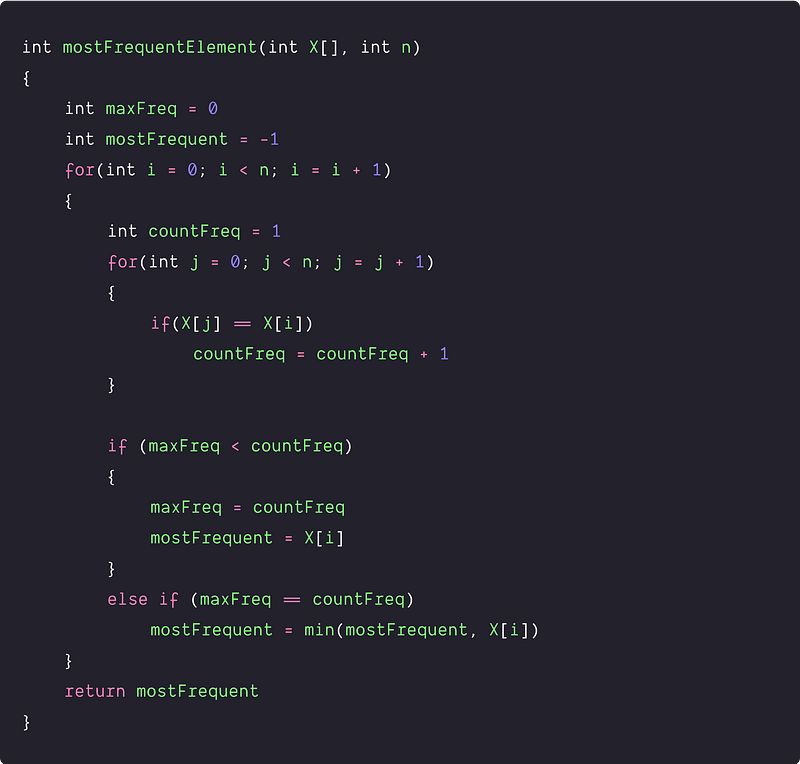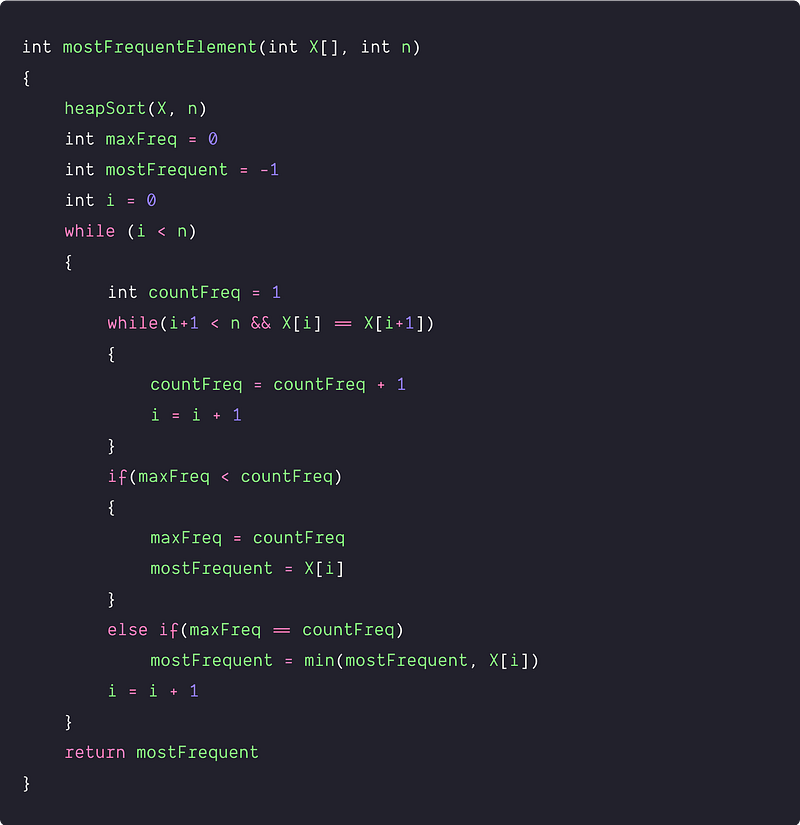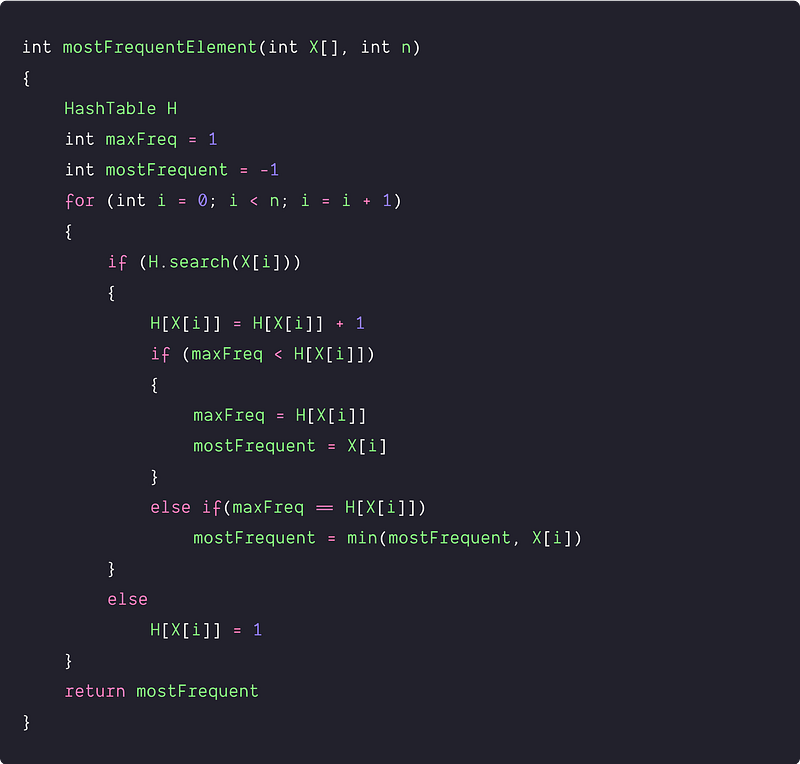# Find most frequent element in an array

Key takeaway: An excellent-searching problem to learn problem-solving and time complexity optimization using sorting and hash table.

### Let’s understand the problem

Given an array X[] of size n, write a program to find the most frequent element in the array, i.e. the element which occurs the maximum number of times.

• It is assumed that at least one element is repeated.
• If there are multiple elements with maximum frequency, return the smallest of them.

Example 1

Input: X[] = [2, 12, 1, 2, 8, 2, 2, 1, 8, 2], Output: 2

Explanation: 2 is most frequent element which appears 4 times.

Example 2

Input: X[] = [1, 9, 1, 1, 2], Output: 1

Explanation: 1 is a single repeated element that appears 3 times.

Example 3

Input: X[] = [1, 9, 2, 1, 2], Output: 1

Explanation: 1 and 2 are repeated two times, then we return the smallest of them i.e. 1.

### Discussed solution approaches

• A brute force approach using nested loops
• Using sorting and linear scan
• Using a hash table to store the frequency count

### A Brute force approach using nested loops

Solution Idea and Steps

The basic idea would be to count the occurrences of each element and return the element with the maximum number of occurrences. We can use two nested loops: pick each element using the outer loop and scan the entire array to count the frequency using the inner loop. Here are solutions steps:

1. We initialize two variables outside the nested loops: maxFreq to track the maximum frequency and mostFrequent to track the most frequent element. maxFreq = 0, mostFrequent = -1.
2. We run an outer loop from i = 0 to n-1 and an inner loop from j = 0 to n-1 to count the frequency. Before starting the inner loop, we also initialize a variable countFreq to track the frequency count of each element i.e. countFreq = 1.

• Inside the inner loop, when we found X[i] = X[j], we increase the value of countFreq by one and move to the next iteration of the inner loop.
• By end of the inner loop, If(maxFreq < countFreq), then we found an element X[i] with a frequency greater than maximum frequency count till that point. So we update maxFreq with countFreq and mostFrequent with X[i].
• If (countFreq == maxFreq), then we update the mostFrequent with the minimum of mostFrquent and X[i] i.e. min(mostFrequent, X[i]). Think!
3. By the end of the nested loops, we return the value stored in mostFrequent.

Solution PseudocodeTime and space complexity analysis

We are running two nested loops and performing an O(1) operation at each iteration. So time complexity = n*n*O(1) = O(n^2). We are using a constant number of extra variables, so space Complexity = O(1)

### Using sorting and single scan

Solution Idea

Now the critical question is: how do we improve the time complexity? Searching is the essential operation in the problem, so can we improve the time complexity of the searching? Let's think!

If we sort the array, then all the duplicate elements will get placed adjacent to each other. We can easily scan the array, count the frequency of each element and return the element with max frequency. Compared with the above approach, this ensures that frequency is calculated only once for each unique element.

Solution Steps

1. We first sort the array using an efficient O(nlogn) sorting algorithm like merge sort, heap sort, or quicksort. Suppose we are using heap sort, which works efficiently in place.
2. We initialize two variables: maxFreq to track the maximum frequency and mostFrequent to track the most frequent element. maxFreq = 0, mostFrequent = -1.
3. Now we scan the sorted array using a loop till i < n. Inside the loop, we initialize a variable countFreq to track the frequency count i.e. countFreq = 1.

• We start from the first element (i =0) and search its consecutive occurrences using a loop till X[i] = X[i + 1]. In other words, the loop will end when X[i] != X[i+1], and in such a situation, we have moved to the next unique element in the sorted array. During this process, we increase countFreq and i by 1.
• After the inner loop, if(maxFreq < countFreq), then we found an element X[i] with a frequency greater than maximum frequency count till that point. So we update maxFreq with countFreq and mostFrequent with X[i]. If(countFreq == maxFreq) then we update the mostFrequent with the minimum of mostFrquent and X[i].
• Now we go to the next iteration of the outer loop and repeat the same process for the next unique element available at index i.
4. By the end of the outer loop, we return the value stored in mostFrequent.

Solution PseudocodeTime and space complexity analysis

If we observe the nested loops, outer loops pick the unique elements, and the inner loop counts the frequency of that element. So we are accessing each element only once and performing O(1) operation (Think!). So the time complexity of the nested loops = n*O(1) = O(n).

Overall Time complexity = Time complexity of the heap sort + Time complexity of the nested loops = O(nlogn) + O(n) = O(n). Space Complexity = O(n), because heap sort works in-place and we are using some constant number of variables.

### Using a hash table to store the frequency count

Solution Idea and Steps

Again, how do we further improve the time complexity? Can we use a hash table to perform searching efficiently? Hash table performs basic dictionary operations like insertion, deletion, and searching operation in O(1) average. So here is another idea:

• We create a hash table H to store the elements, and their frequency counts as key-value pairs.
• Now we scan the array to check if the value associated with any element exists in the Hash Table or not. If it exists, we increment the frequency count(value) of the element(key) by 1. If not, store the frequency count of that element by 1.
• During the iteration, we also store the most frequent element and its frequency count in the variable mostFrequent and maxFreq, respectively. We update the value of these variables if we found the stored frequency count of any element greater than the current max frequency count.
• And finally, return the most frequent element found i.e. return mostFrequent.

Solution PseudocodeTime and space complexity analysis

We are doing a single scan of the array and, at each iteration, performing constant operations. In other words, we are searching for each element once and inserting/updating it’s once. So, time Complexity = n*O(1) = O(n). Space Complexity = O(n) for storing elements in the Hash Table.

### Critical ideas to think!

• Can we use BST for the solution? What would be the time complexity?
• Can we solve this problem using some other approach?
• How can we optimize the brute force approach further? How do we ensure that the frequency is calculated only once for each unique element?
• How can we optimize the 2nd approach further? Would it be possible to use the binary search after sorting to find the most frequent element?
• What if all elements were unique? What changes will you make?

### Comparisons of time and space complexities

• Using nested loops: Time = O(n^2), Space = O(1)
• Using sorting and linear scan: Time = O(nlogn), Space = O(1)
• Using a Hash Table: Time = O(n), Space = O(n)

### Suggested coding problems to practice

• Find the most frequent word in a sentence
• Sort characters by frequency
• Find the frequency of all words in a sentence
• Find the least frequent element in the array
• Find the top k frequent elements in the array

Enjoy learning, Enjoy algorithms!

Subscribe to get well-designed content on data structures and algorithms, machine learning, system design, oops, and mathematics. enjoy learning!

### We Welcome Doubts and Feedback!